Do we know why the monster exists and why there’s moonshine around it?

The answer depends on whether or not you believe that vertex operator algebras are natural, elegant and inescapable objects.

the monster

Simple groups often arise from symmetries of exceptionally nice mathematical objects.

The smallest of them all, $A_5$, gives us the rotation symmetries of the icosahedron. The next one, Klein’s simple group $L_2(7)$, comes from the Klein quartic.

The smallest sporadic groups, the Mathieu groups, come from Steiner systems, and the Conway groups from the 24-dimensional Leech lattice.

What about the largest sporadic simple, the monster $\mathbb{M}$?

In his paper What is … the monster? Richard Borcherds writes (among other characterisations of $\mathbb{M}$):

“3. It is the automorphism group of the monster vertex algebra. (This is probably the best answer.)”

But, even Borcherds adds:

“Unfortunately none of these definitions is completely satisfactory. At the moment all constructions of the algebraic structures above seem artificial; they are constructed as sums of two or more apparently unrelated spaces, and it takes a lot of effort to define the algebraic structure on the sum of these spaces and to check that the monster acts on the resulting structure.
It is still an open problem to find a really simple and natural construction of the monster vertex algebra.

Here’s 2 minutes of John Conway on the “one thing” he really wants to know before he dies: why the monster group exists.

moonshine

Moonshine started off with McKay’s observation that 196884 (the first coefficient in the normalized j-function) is the sum 1+196883 of the dimensions of the two smallest simple representations of $\mathbb{M}$.

Soon it was realised that every conjugacy class of the monster has a genus zero group (or ‘moonshine group’) associated to it.

Borcherds proved the ‘monstrous moonshine conjectures’ asserting that the associated main modular function of such a group is the character series of the action of the element on the monster vertex algebra.

Here’s Borcherds’ ICM talk in Berlin on this: What is … Moonshine?.

Once again, the monster vertex algebra appears to be the final answer.

However, in characterising the 171 moonshine groups among all possible genus zero groups one has proved that they are all of the form:

(ii) : $(n|h)+e,g,\dots$

In his book Moonshine beyond the Monster, Terry Gannon writes:

“We now understand the significance, in the VOA or CFT framework, of transformations in $SL_2(\mathbb{Z})$, but (ii) emphasises that many modular transformations relevant to Moonshine are more general (called the Atkin-Lehner involutions).
Monstrous moonshine will remain mysterious until we can understand its Atkin-Lehner symmetries.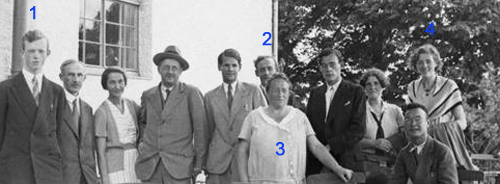For the better part of the 30ties, Ernst Witt (1) did hang out with the rest of the ‘Noetherknaben’, the group of young mathematicians around Emmy Noether (3) in Gottingen.

In 1934 Witt became Helmut Hasse‘s assistent in Gottingen, where he qualified as a university lecturer in 1936. By 1938 he has made enough of a name for himself to be offered a lecturer position in Hamburg and soon became an associate professor, the down-graded position held by Emil Artin (2) until he was forced to emigrate in 1937.

A former fellow student of him in Gottingen, Erna Bannow (4), had gone earlier to Hamburg to work with Artin. She continued her studies with Witt and finished her Ph.D. in 1939. In 1940 Erna Bannow and Witt married.

So, life was smiling on Ernst Witt that sunday january 28th 1940, both professionally and personally. There was just one cloud on the horizon, and a rather menacing one. He was called up by the Wehrmacht and knew he had to enter service in february. For all he knew, he was spending the last week-end with his future wife… (later in february 1940, Blaschke helped him to defer his military service by one year).

Still, he desperately wanted to finish his paper before entering the army, so he spend most of that week-end going through the final version and submitted it on monday, as the published paper shows.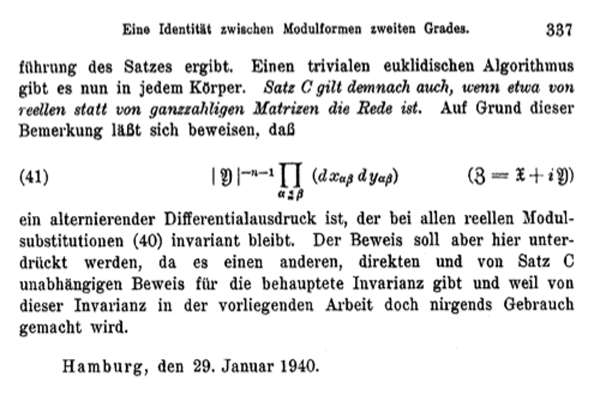In the 70ties, Witt suddenly claimed he did discover the Leech lattice ${\Lambda}$ that sunday. Last time we have seen that the only written evidence for Witt’s claim is one sentence in his 1941-paper Eine Identität zwischen Modulformen zweiten Grades. “Bei dem Versuch, eine Form aus einer solchen Klassen wirklich anzugeben, fand ich mehr als 10 verschiedene Klassen in ${\Gamma_{24}}$.”

But then, why didn’t Witt include more details of this sensational lattice in his paper?

Ina Kersten recalls on page 328 of Witt’s collected papers : “In his colloquium talk “Gitter und Mathieu-Gruppen” in Hamburg on January 27, 1970, Witt said that in 1938, he had found nine lattices in ${\Gamma_{24}}$ and that later on January 28, 1940, while studying the Steiner system ${S(5,8,24)}$, he had found two additional lattices ${M}$ and ${\Lambda}$ in ${\Gamma_{24}}$. He continued saying that he had then given up the tedious investigation of ${\Gamma_{24}}$ because of the surprisingly low contribution

$\displaystyle | Aut(\Lambda) |^{-1} < 10^{-18}$

to the Minkowski density and that he had consented himself with a short note on page 324 in his 1941 paper.”

In the last sentence he refers to the fact that the sum of the inverse orders of the automorphism groups of all even unimodular lattices of a given dimension is a fixed rational number, the Minkowski-Siegel mass constant. In dimension 24 this constant is

$\displaystyle \sum_{L} \frac{1}{| Aut(L) |} = \frac {1027637932586061520960267}{129477933340026851560636148613120000000} \approx 7.937 \times 10^{-15}$

That is, Witt was disappointed by the low contribution of the Leech lattice to the total constant and concluded that there might be thousands of new even 24-dimensional unimodular lattices out there, and dropped the problem.

If true, the story gets even better : not only claims Witt to have found the lattices ${A_1^{24}=M}$ and ${\Lambda}$, but also enough information on the Leech lattice in order to compute the order of its automorphism group ${Aut(\Lambda)}$, aka the Conway group ${Co_0 = .0}$ the dotto-group!

Is this possible? Well fortunately, the difficulties one encounters when trying to compute the order of the automorphism group of the Leech lattice from scratch, is one of the better documented mathematical stories around.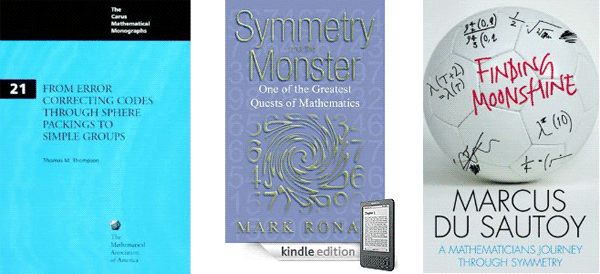The books From Error-Correcting Codes through Sphere Packings to Simple Groups by Thomas Thompson, Symmetry and the monster by Mark Ronan, and Finding moonshine by Marcus du Sautoy tell the story in minute detail.

It took John Conway 12 hours on a 1968 saturday in Cambridge to compute the order of the dotto group, using the knowledge of Leech and McKay on the properties of the Leech lattice and with considerable help offered by John Thompson via telephone.

But then, John Conway is one of the fastest mathematicians the world has known. The prologue of his book On numbers and games begins with : “Just over a quarter of a century ago, for seven consecutive days I sat down and typed from 8:30 am until midnight, with just an hour for lunch, and ever since have described this book as “having been written in a week”.”

Conway may have written a book in one week, Ernst Witt did complete his entire Ph.D. in just one week! In a letter of August 1933, his sister told her parents : “He did not have a thesis topic until July 1, and the thesis was to be submitted by July 7. He did not want to have a topic assigned to him, and when he finally had the idea, he started working day and night, and eventually managed to finish in time.”

So, if someone might have beaten John Conway in fast-computing the dottos order, it may very well have been Witt. Sadly enough, there is a lot of circumstantial evidence to make Witt’s claim highly unlikely.

For starters, psychology. Would you spend your last week-end together with your wife to be before going to war performing an horrendous calculation?

Secondly, mathematical breakthroughs often arise from newly found insight. At that time, Witt was also working on his paper on root lattices “Spiegelungsgrupen and Aufzähling halbeinfacher Liescher Ringe” which he eventually submitted in january 1941. Contained in that paper is what we know as Witt’s lemma which tells us that for any integral lattice the sublattice generated by vectors of norms 1 and 2 is a direct sum of root lattices.

This leads to the trick of trying to construct unimodular lattices by starting with a direct sum of root lattices and ‘adding glue’. Although this gluing-method was introduced by Kneser as late as 1967, Witt must have been aware of it as his 16-dimensional lattice ${D_{16}^+}$ is constructed this way.

If Witt wanted to construct new 24-dimensional even unimodular lattices in 1940, it would be natural for him to start off with direct sums of root lattices and trying to add vectors to them until he got what he was after. Now, all of the Niemeier-lattices are constructed this way, except for the Leech lattice!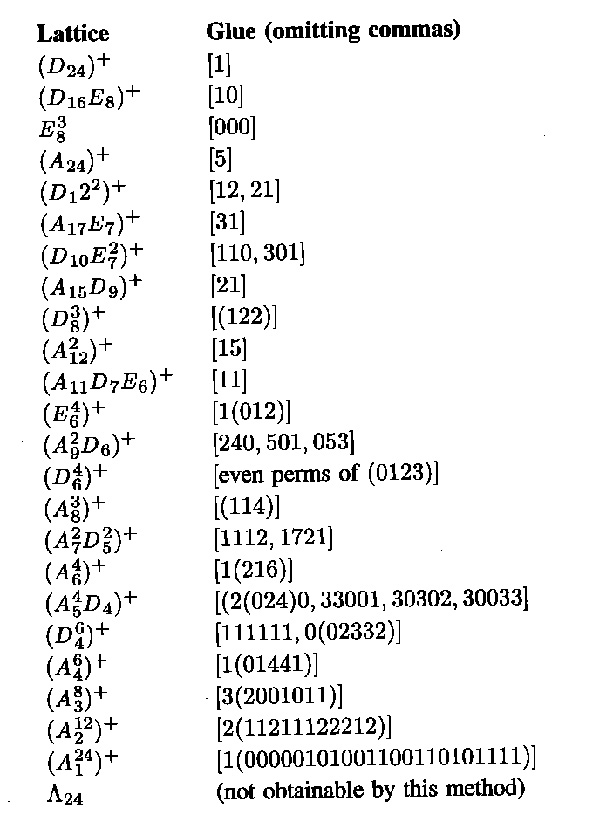I’m far from an expert on the Niemeier lattices but I would say that Witt definitely knew of the existence of ${D_{24}^+}$, ${E_8^3}$ and ${A_{24}^+}$ and that it is quite likely he also constructed ${(D_{16}E_8)^+, (D_{12}^2)^+, (A_{12}^2)^+, (D_8^3)^+}$ and possibly ${(A_{17}E_7)^+}$ and ${(A_{15}D_9)^+}$. I’d rate it far more likely Witt constructed another two such lattices on sunday january 28th 1940, rather than discovering the Leech lattice.

Finally, wouldn’t it be natural for him to include a remark, in his 1941 paper on root lattices, that not every even unimodular lattices can be obtained from sums of root lattices by adding glue, the Leech lattice being the minimal counter-example?

If it is true he was playing around with the Steiner systems that sunday, it would still be a pretty good story he discovered the lattices ${(A_2^{12})^+}$ and ${(A_1^{24})^+}$, for this would mean he discovered the Golay codes in the process!

Which brings us to our next question : who discovered the Golay code?

The Leech lattice was, according to wikipedia, ‘originally discovered by Ernst Witt in 1940, but he did not publish his discovery’ and it ‘was later re-discovered in 1965 by John Leech’. However, there is very little evidence to support this claim.

The facts

What is certain is that John Leech discovered in 1965 an amazingly dense 24-dimensional lattice ${\Lambda}$ having the property that unit balls around the lattice points touch, each one of them having exactly 196560 neighbors. The paper ‘Notes on sphere packings’ appeared in 1967 in the Canad. J. Math. 19, 251-267.

Compare this to the optimal method to place pennies on a table, leading to the hexagonal tiling, each penny touching exactly 6 others. Similarly, in dimension 8 the densest packing is the E8 lattice in which every unit ball has exactly 240 neighbors.

The Leech lattice ${\Lambda}$ can be characterized as the unique unimodular positive definite even lattice such that the length of any non-zero vector is at least two.

The list of all positive definite even unimodular lattices, ${\Gamma_{24}}$, in dimension 24 was classified later by Hans-Volker Niemeier and are now known as the 24 Niemeier lattices.For the chronology below it is perhaps useful to note that, whereas Niemeier’s paper did appear in 1973, it was submitted april 5th 1971 and is just a minor rewrite of Niemeier’s Ph.D. “Definite quadratische Formen der Dimension 24 und Diskriminante 1” obtained in 1968 from the University of Göttingen with advisor Martin Kneser.

The claim

On page 328 of Ernst Witt’s Collected Papers Ina Kersten recalls that Witt gave a colloquium talk on January 27, 1970 in Hamburg entitled “Gitter und Mathieu-Gruppen” (Lattices and Mathieu-groups). In this talk Witt claimed to have found nine lattices in ${\Gamma_{24}}$ as far back as 1938 and that on January 28, 1940 he found two additional lattices ${M}$ and ${\Lambda}$ while studying the Steiner system ${S(5,8,24)}$.

On page 329 of the collected papers is a scan of the abstract Witt wrote in the colloquium book in Bielefeld where he gave a talk “Uber einige unimodularen Gitter” (On certain unimodular lattices) on January 28, 1972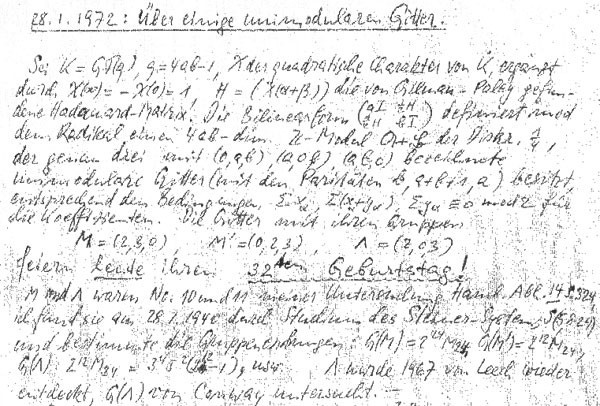Here, Witt claims that he found three new lattices in ${\Gamma_{24}}$ on January 28, 1940 as the lattices ${M}$, ${M’}$ and ${\Lambda}$ ‘feiern heute ihren 32sten Gebursttag!’ (celebrate today their 32nd birthday).

He goes on telling that the lattices ${M}$ and ${\Lambda}$ were number 10 and 11 in his list of lattices in ${\Gamma_{24}}$ in his paper “Eine Identität zwischen Modulformen zweiten Grades” in the Abh. Math. Sem. Univ. Hamburg 14 (1941) 323-337 and he refers in particular to page 324 of that paper.

He further claims that he computed the orders of their automorphism groups and writes that ${\Lambda}$ ‘wurde 1967 von Leech wieder-entdeckt’ (was re-discovered by Leech in 1967) and that its automorphism group ${G(\Lambda)}$ was studied by John Conway. Recall that Conway’s investigations of the automorphism group of the Leech lattice led to the discovery of three new sporadic groups, the Conway groups ${Co_1,Co_2}$ and ${Co_3}$.

However, Witt’s 1941-paper does not contain a numbered list of 24-dimensional lattices. In fact, apart from ${E_8+E_8+E_8}$ is does not contain a single lattice in ${\Gamma_{24}}$. The only relevant paragraph is indeed on page 324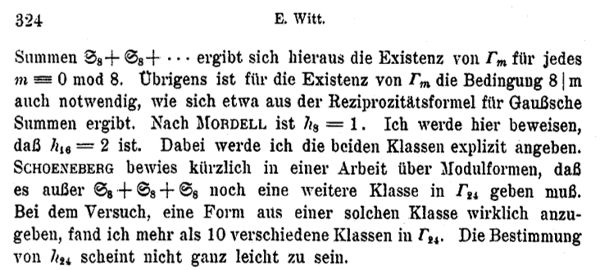He observes that Mordell already proved that there is just one lattice in ${\Gamma_8}$ (the ${E_8}$-lattice) and that the main result of his paper is to prove that there are precisely two even unimodular 16-dimensional lattices : ${E_8+E_8}$ and another lattice, now usually called the 16-dimensional Witt-lattice.

He then goes on to observe that Schoeneberg knew that ${\# \Gamma_{24} > 1}$ and so there must be more lattices than ${E_8+E_8+E_8}$ in ${\Gamma_{24}}$. Witt concludes with : “In my attempt to find such a lattice, I discovered more than 10 lattices in ${\Gamma_{24}}$. The determination of ${\# \Gamma_{24}}$ does not seem to be entirely trivial.”

Hence, it is fair to assume that by 1940 Ernst Witt had discovered at least 11 of the 24 Niemeier lattices. Whether the Leech lattice was indeed lattice 11 on the list is anybody’s guess.

Next time we will look more closely into the historical context of Witt’s 1941 paper.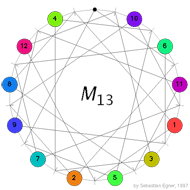Conway’s puzzle M(13) involves the 13 points and 13 lines of $\mathbb{P}^2(\mathbb{F}_3)$. On all but one point numbered counters are placed holding the numbers 1,…,12 and a move involves interchanging one counter and the ‘hole’ (the unique point having no counter) and interchanging the counters on the two other points of the line determined by the first two points. In the picture on the left, the lines are respresented by dashes around the circle in between two counters and the points lying on this line are those that connect to the dash either via a direct line or directly via the circle. In the first part we saw that the group of all reachable positions in Conway’s M(13) puzzle having the hole at the top positions contains the sporadic simple Mathieu group $M_{12}$ as a subgroup. To see the reverse inclusion we have to recall the definition of the ternary Golay code named in honour of the Swiss engineer Marcel Golay who discovered in 1949 the binary Golay code that we will encounter _later on_.

The ternary Golay code $\mathcal{C}_{12}$ is a six-dimenional subspace in $\mathbb{F}_3^{\oplus 12}$ and is spanned by its codewords of weight six (the Hamming distance of $\mathcal{C}_{12}$ whence it is a two-error correcting code). There are $264 = 2 \times 132$ weight six codewords and they can be obtained from the 132 hexads, we encountered before as the winning positions of Mathieu’s blackjack, by replacing the stars by signs + or – using the following rules. By a tet (from tetracodeword) we mean a 3×4 array having 4 +-signs indicating the row-positions of a tetracodeword. For example

$~\begin{array}{|c|ccc|} \hline & + & & \\ + & & + & \\ & & & + \\ \hline + & 0 & + & – \end{array}$ is the tet corresponding to the bottom-tetracodeword. $\begin{array}{|c|ccc|} \hline & + & & \\ & + & & \\ & + & & \\ \hline & & & \end{array}$ A col is an array having +-signs along one of the four columns. The signed hexads will now be the hexads that can be written as $\mathbb{F}_3$ vectors as (depending on the column-distributions of the stars in the hexad indicated between brackets)

$col-col~(3^20^2)\qquad \pm(col+tet)~(31^3) \qquad tet-tet~(2^30) \qquad \pm(col+col-tet)~(2^21^2)$

For example, the hexad on the right has column-distribution $2^30$ so its signed versions are of the form tet-tet. The two tetracodewords must have the same digit (-) at place four (so that they cancel and leave an empty column). It is then easy to determine these two tetracodewords giving the signed hexad (together with its negative, obtained by replacing the order of the two codewords)

$\begin{array}{|c|ccc|} \hline \ast & \ast & & \\ \ast & & \ast & \\ & \ast & \ast & \\ \hline – & + & 0 & – \end{array}$ signed as
$\begin{array}{|c|ccc|} \hline + & & & \\ & & & \\ & + & + & + \\ \hline 0 & – & – & – \end{array} – \begin{array}{|c|ccc|} \hline & + & & \\ + & & + & \\ & & & + \\ \hline + & 0 & + & – \end{array} = \begin{array}{|c|ccc|} \hline + & – & & \\ – & & – & \\ & + & + & \\ \hline – & + & 0 & – \end{array}$

and similarly for the other cases. As Conway&Sloane remark ‘This is one of many cases when the process is easier performed than described’.

We have an order two operation mapping a signed hexad to its negative and as these codewords span the Golay code, this determines an order two automorphism of $\mathcal{C}_{12}$. Further, forgetting about signs, we get the Steiner-system S(5,6,12) of hexads for which the automorphism group is $M_{12}$ hence the automorphism group op the ternary Golay code is $2.M_{12}$, the unique nonsplit central extension of $M_{12}$.

Right, but what is the connection between the Golay code and Conway’s M(13)-puzzle which is played with points and lines in the projective plane $\mathbb{P}^2(\mathbb{F}_3)$? There are 13 points $\mathcal{P}$ so let us consider a 13-dimensional vectorspace $X=\mathbb{F}_3^{\oplus 13}$ with basis $x_p~:~p \in \mathcal{P}$. That is a vector in X is of the form $\vec{v}=\sum_p v_px_p$ and consider the ‘usual’ scalar product $\vec{v}.\vec{w} = \sum_p v_pw_p$ on X. Next, we bring in the lines in $\mathbb{P}^2(\mathbb{F}_3)$.

For each of the 13 lines l consider the vector $\vec{l} = \sum_{p \in l} x_p$ with support the four points lying on l and let $\mathcal{C}$ be the subspace (code) of X spanned by the thirteen vectors $\vec{l}$. Vectors $\vec{c},\vec{d} \in \mathcal{C}$ satisfy the remarkable identity $\vec{c}.\vec{d} = (\sum_p c_p)(\sum_p d_p)$. Indeed, both sides are bilinear in $\vec{c},\vec{d}$ so it suffices to check teh identity for two line-vectors $\vec{l},\vec{m}$. The right hand side is then 4.4=16=1 mod 3 which equals the left hand side as two lines either intersect in one point or are equal (and hence have 4 points in common). The identity applied to $\vec{c}=\vec{d}$ gives us (note that the squares in $\mathbb{F}_3$ are {0,1}) information about the weight (that is, the number of non-zero digits) of codewords in $\mathcal{C}$

$wt(\vec{c})~mod(3) = \sum_p c_p^2 = (\sum_p c_p)^2 \in \{ 0,1 \}$

Let $\mathcal{C}’$ be the collection of $\vec{c} \in \mathcal{C}$ of weight zero (modulo 3) then one can verify that $\mathcal{C}’$ is the orthogonal complement of $\mathcal{C}$ with respect to the scalar product and that the dimension of $\mathcal{C}$ is seven whereas that of $\mathcal{C}’$ is six.
Now, let for a point p be $\mathcal{G}_p$ the restriction of

$\mathcal{C}_p = \{ c \in \mathcal{C}~|~c_p = – \sum_{q \in \mathcal{P}} c_q \}$

to the coordinates of $\mathcal{P} – \{ p \}$, then $\mathcal{G}_p$ is clearly a six dimensional code in a 12-dimensional space. A bit more work shows that $\mathcal{G}_p$ is a self-dual code with minimal weight greater or equal to six, whence it must be the ternary Golay code! Now we are nearly done. _Next time_ we will introduce a reversi-version of M(13) and use the above facts to deduce that the basic group of the Mathieu-groupoid indeed is the sporadic simple group $M_{12}$.

References

Robert L. Griess, “Twelve sporadic groups” chp. 7 ‘The ternary Golay code and $2.M_{12}$’

John H. Conway and N. J.A. Sloane, “Sphere packings, lattices and groups” chp 11 ‘The Golay codes and the Mathieu groups’

John H. Conway, Noam D. Elkies and Jeremy L. Martin, ‘The Mathieu group $M_{12}$ and its pseudogroup extension $M_{13}$’ arXiv:math.GR/0508630

If you only tune in now, you might want to have a look at the definition of Mathieu’s blackjack and the first part of the proof of the Conway-Ryba winning strategy involving the Steiner system S(5,6,12) and the Mathieu sporadic group $M_{12}$.

We’re trying to disprove the existence of misfits, that is, of non-hexad positions having a total value of at least 21 such that every move to a hexad would increase the total value. So far, we succeeded in showing that such a misfit must have the patern

$\begin{array}{|c|ccc|} \hline 6 & III & \ast & 9 \\ 5 & II & 7 & . \\ IV & I & 8 & . \\ \hline & & & \end{array}$

That is, a misfit must contain the 0-card (queen) and cannot contain the 10 or 11(jack) and must contain 3 of the four Romans. Now we will see that a misfit also contains precisely one of {5,6} (and consequently also exactly one card from {7,8,9}). To start, it is clear that it cannot contain BOTH 5 and 6 (then its total value can be at most 20). So we have to disprove that a misfit can miss {5,6} entirely (and so the two remaining cards (apart from the zero and the three Romans) must all belong to {7,8,9}).

Lets assume the misfit misses 5 and 6 and does not contain 9. Then, it must contain 4 (otherwise, its column-distribution would be (0,3,3,0) and it would be a hexad). There are just three such positions possible

$\begin{array}{|c|ccc|} \hline . & \ast & \ast & . \\ . & \ast & \ast & . \\ \ast & . & \ast & . \\ \hline – & – & ? & ? \end{array}$ $\begin{array}{|c|ccc|} \hline . & \ast & \ast & . \\ . & . & \ast & . \\ \ast & \ast & \ast & . \\ \hline – & + & ? & ? \end{array}$ $\begin{array}{|c|ccc|} \hline . & . & \ast & . \\ . & \ast & \ast & . \\ \ast & \ast & \ast & . \\ \hline – & 0 & ? & ? \end{array}$

Neither of these can be misfits though. In the first one, there is an 8->5 move to a hexad of smaller total value (in the second a 7->5 move and in the third a 7->6 move). Right, so the 9 card must belong to a misfit. Assume it does not contain the 4-card, then part of the misfit looks like (with either a 7- or an 8-card added)

$\begin{array}{|c|ccc|} \hline . & \ast & \ast & \ast \\ . & \ast & ? & . \\ . & \ast & ? & . \\ \hline & & & \end{array}$ contained in the unique hexad $\begin{array}{|c|ccc|} \hline \ast & \ast & \ast & \ast \\ . & \ast & & . \\ . & \ast & & . \\ \hline & & & \end{array}$

Either way the moves 7->6 or 8->6 decrease the total value, so it cannot be a misfit. Therefore, a misfit must contain both the 4- and 9-card. So it is of the form on the left below

$\begin{array}{|c|ccc|} \hline . & ? & \ast & \ast \\ . & ? & ? & . \\ \ast & ? & ? & . \\ \hline & & & \end{array}$ $\begin{array}{|c|ccc|} \hline . & . & \ast & . \\ . & \ast & \ast & \ast \\ \ast & \ast & . & . \\ \hline – & 0 & – & + \end{array}$ $\begin{array}{|c|ccc|} \hline . & . & \ast & \ast \\ . & \ast & \ast & . \\ \ast & \ast & . & . \\ \hline & & & \end{array}$

If this is a genuine misfit only the move 9->10 to a hexad is possible (the move 9->11 is not possible as all BUT ONE of {0,1,2,3,4} is contained in the misfit). Now, the only hexad containing 0,4,10 and 2 from {1,2,3} is in the middle, giving us what the misfit must look like before the move, on the right. Finally, this cannot be a misfit as the move 7->5 decreases the total value.

That is, we have proved the claim that a misfit must contain one of {5,6} and one of {7,8,9}. Right, now we can deliver the elegant finishing line of the Kahane-Ryba proof. A misfit must contain 0 and three among {1,2,3,4} (let us call the missing card s), one of $5+\epsilon$ with $0 \leq \epsilon \leq 1$ and one of $7+\delta$ with $0 \leq \delta \leq 2$. Then, the total value of the misfit is

$~(0+1+2+3+4-s)+(5+\epsilon)+(7+\delta)=21+(1+\delta+\epsilon-s)$

So, if this value is strictly greater than 21 (and we will see in a moment is has to be if it is at least 21) then we deduce that $s < 1 + \delta + \epsilon \leq 4$. Therefore $1+\delta+\epsilon$ belongs to the misfit. But then the move $1+\delta \epsilon \rightarrow s$ moves the misfit to a 6-tuple with total value 21 and hence (as we see in a moment) must be a hexad and hence this is a decreasing move! So, finally, there are no misfits!

Hence, from every non-hexad pile of total value at least 21 we have a legal move to a hexad. Because the other player cannot move from an hexad to another hexad we are done with our strategy provided we can show (a) that the total value of any hexad is at least 21 and (b) that ALL 6-piles of total value 21 are hexads. As there are only 132 hexads it is easy enough to have their sum-distribution. Here it is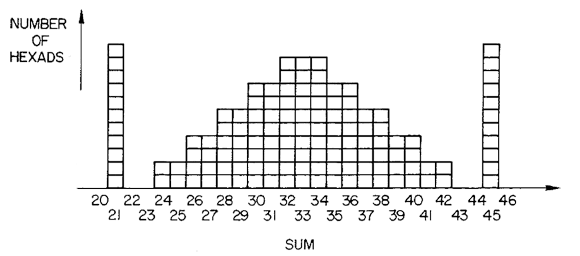That is, (a) is proved by inspection and we see that there are 11 hexads of sum 21 (the light hexads in Conway-speak) and there are only 11 ways to get 21 as a sum of 6 distinct numbers from {0,1,..,11} so (b) follows. Btw. the obvious symmetry of the sum-distribution is another consequence of the duality t->11-t discussed briefly at the end of part 2.

Clearly, I’d rather have conceptual proofs for all these facts and briefly tried my hand. Luckily I did spot the following phrase on page 326 of Conway-Sloane (discussing the above distribution) :

“It will not be easy to explain all the above observations. They are certainly connected with hyperbolic geometry and with the ‘hole’ structure of the Leech lattice.”

So, I’d better leave it at this…

References

Joseph Kahane and Alexander J. Ryba, “The hexad game

John H. Conway and N. J.A. Sloane, “Sphere packings, Lattices and Groups” chp. 11 ‘The Golay codes and the Mathieu groups’

Can't find what you're looking for? Try refining your search: